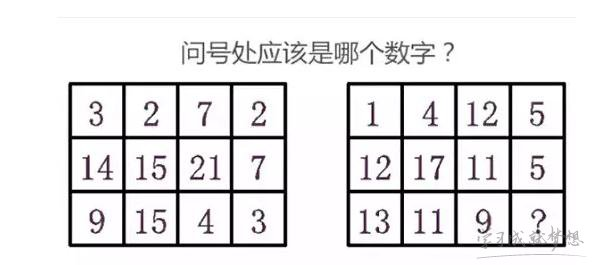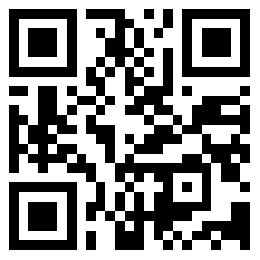# 三道思维题练习

（1）看图分析数字【答案】：2

解析：左边表格上下的数字相加等于右边表格中间的数字;右边表格上下的数字相加等于左边表格中间的数字。

(2)挂钟

【答案】:

结果：2×(1+1+2+1+3+1+4+1+5+1+6+1+7+1+8+1+9+1+10+1+11+1+12+1)=180下

(3)猫抓老鼠数学智力题答案

答案解析:

• 上一篇: 没有了
• 下一篇: 推理分析智力问答

### 热门阅读微信扫码关注
随时手机看书